Internet of Things and Cloud Computing
Volume 3, Issue 3, October 2015, Pages: 48-58

Research on Innovating and Applying Fuzzy Logic for Routing Technique in Service Oriented Routing

Nguyen Thanh Long1, Nguyen Duc Thuy2, Pham Huy Hoang3, *

1Informatic Center of Ha Noi Telecommunications, HoanKiem, HaNoi, VietNam

2Post and Telecommunications Institute, HaNoi, VietNam

3HaNoi University of Science Technology, HaNoi, VietNam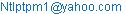(N. T. Long)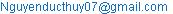(N. D. Thuy)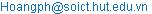(P. H. Hoang)

Nguyen Thanh Long, Nguyen Duc Thuy, Pham Huy Hoang. Research on Innovating and Applying Fuzzy Logic for Routing Technique in Service Oriented Routing. Internet of Things and Cloud Computing. Vol. 3, No. 3, 2015, pp. 48-58. doi: 10.11648/j.iotcc.s.2015030601.16

Abstract: Mobile Ad-hoc Network (Manet) consists of a set of network nodes which change, not based on an existing network infrastructure as well as the centralized control. Network nodes usually have limited energy, bandwidth limitations, so find a way to transfer packets in the network is very important. To minimize packet loss, efficient bandwidth utilization at the node requires routing protocol must be compact, efficient, accurate. Fuzzy logic is a logical field studies evaluating the accuracy of the results based on the approximation of the components involved, make decisions based on many factors relative accuracy based on experimental or mathematical proof. Each component of the logical expression is usually belong to one set of defined unclear (fuzzy set). In this paper, We give some researchs applied fuzzy logic in assessing the network routing and bandwidth management on the basis of a number of existing studies.

Keywords: Routing, Fuzzy, Logic, Multi-Path, Bandwidth, Energy, Manet, Capacity

1. Overview

1.1. Mobile Ad Hoc NETwork (MANET)

MANET is formed from many network nodes which regularly move and change quickly, so most of calculated results for MANET have certain correctness level. Therefore, the application of fuzzy logic to calculate, estimate the components from which to calculate, evaluate, estimate the route bandwidth and multi-paths routing for MANET is very important. Network node in MANET often has limited energy. So finding routes use less power, large bandwidth are important, help reduce energy consumption, transmission delay.

1.2. Fuzzy Logic

1.2.1. Ordinary Logic

Logic usually only interested in two absolute values (true or false) and following two assumptions: i) the members of the set, with an element and one any set of some elements, then this element can belong to this set or the complement of that set; ii) the law of excluding mediate element, confirming an element can not both belong to a set and the complement of it. So the traditional logic can not support the inference on the information that is vague, unclear.

1.2.2. Fuzzy logic

The concept of fuzzy logic: To overcome the shortcomings of the traditional logic, Lotfi Zadeh has proposed one new theory of logic called fuzzy logic. Zadeh's theory represented the fuzzy or inaccuracy of logical clauses in quantitative way by giving a set of membership functions, given function’s value in the range [0,1] . With S is a set, x is one element of the set, a fuzzy subset F of S is defined by one membership function μF(x) measuring the level that x belongs to F, with condition 0 ≤ μF(x) ≤ 1: i) with μF(x) = 0: x is completely not belong to F; ii) with μF(x) = 1: x completely belongs F; iii) μF(x) =: F is called "brittle" set.

The correctness of the logical expression is based on a set of rules gotten from experts or mathematical proof.

Fuzzy logic are often used in decision support systems , used to approximate the function. Fuzzy logic system used to model or perform continuous function. Quality assessment of fuzzy expression depends on the quality of the law.

2. Related Rsearch

Up to now there are some researchs on QoS routing based on fuzzy logic. In this paper, refer to , transmit a pair of packets RREQ and RREP to detect and get information on routes from a source node to a set of destination nodes hop by hop. Use balanced used energy and remaining power routing protocol based on fuzzy logic . This protocol estimates remaining power and required energy to transmitdata for any node along route to decide this node on the route or not. On a destination node, estimate bandwidth of route detected based on un-used time slots of all nodes along the route . On the source node, after receiving all the routes from RREPs in regulated time period, build multicast trees for QoS and guaranteed bandwith routing.

3. Establish Fuzzy Logic System for Assessing Route

3.1. Model of Fuzzy Logic controller (FLC) [5,8]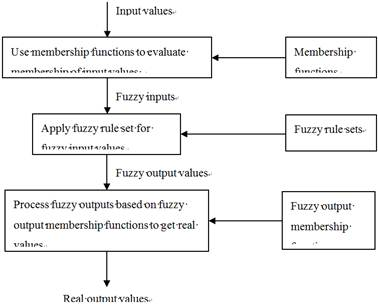Fig. 1. Model of a fuzzy logic controller.

One fuzzy logic system composed of three components: i) Make fuzzy values: this is process that use conversion functions to convert input data into membership values in the range [0,1]; ii) Inference: use inference functions that are based on fuzzy rule sets to get fuzzy result sets; iii) Get real values: in this process that use conversion functions process fuzzy results to get real results based on fuzzy output rule sets.

3.2. Propose Some Parameters to Estimate Route Based on Fuzzy Logic

a)  Remaining power at every node of the route;

b)  Consumption energy at every node to transmit data;

c)  Hop count of the route;

d)  Bandwidth of the route ;

e)  Temperature of the node;

f)   Queue length of the node;

g)  Physical length of the route.

Depending on QoS, these parameters will be combinedto input for afuzzy logic controller or several fuzzy logic controllers to make decisions for estimating route efficiently. Then this decision will be broadcast into network, at every received node, data will be made to be fuzzy in combining with cached data on this node, by the formula:

Fuzzifier_Function (New_Data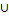Cached_Data)= {Fuzzy_Sets_Data}.

3.3. Use the Pair of Packets RREP to Detect Route 

In this paper, use the pair of packets Route Request(RREQ) and Route Reply (RREP) to detect route and calculate important quantities in every node based on information received in RREQ packet with information cached on this node, complement to this RREQ for relaying this packet to destination nodes.

The quantities need to be calculated depending on specific proposed requirements. In this paper, will analyze some parameters for measuring and defining link bandwidth and remaining power, estimating consumption energy required to transfer data, length of buffer, temperature in every node of the route. These factors will be combined depending on actual requirements to enter fuzzy logic controller for calculating some important parameters to decide route.

When RREQ arrives at a destination node, all received parameters will be put to fuzzy logic controller to make decision whether select this received route or not.

If the route is selected, all information about this route will be transferred to the source node by RREP packet by detected route in backward direction. At each intermediate node on the route that RREP passes on, this route will be cached.

3.4. Algorithm Diagram for Detecting Route and Sending Data Based on Fuzzy Logic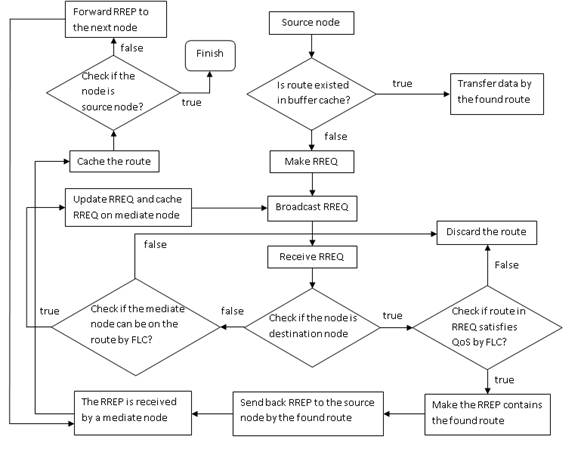Fig. 2. he diagram of the algorithm for finding route satisfies QoS requirements based on fuzzy logic.

Check whether route  in buffer cache or not? If it exists then send data by this found route. If not then it finds route by establishing RREQ packet and broadcast to network. In every immediate node, when receiving a RREQ packet, it checks whether its address is in Destination address list or not:

a)  If it is true, estimate route by fuzzy logic controller;

b)  Otherwise, calculate fuzzy result based on some measured metrics at recent and in the past in some time interval to decide whether transfer RREQ or not.

This model represents general route detecting method in typical network, but in clustered network, detected route will mainly pass on some cluster heads and gateway nodes as analysing in section (4.3). So the route is established fast and reduce cost for finding. Therefore the detecting route is very effectively and lower cost for data transmission of the pair of packages RREQ and RREP.

4. Fuzzy Logic Controller Model for Calculating Parameters to Select Route

4.1. Fuzzy Logic Controller Model

Depend on the pair of packets RREQ and RREP as mentioned above :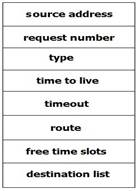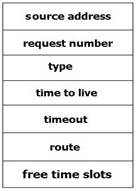Route Request packet       Route Reply packet

Fig. 3. The structure of a pair of RREQ and RREP.

a)        At every mediate node, when it receives a RREQ packet, it will check whether it has received this packet or not: (i) if true it will drop this packet; (ii) otherwise, measure some parameters:

1)  Node’s bandwidth,

2)  Buffer length,

3)  Remaining power,

4)  Required energy to transfer data ,

5)  Distance between this node (N) and previous node which has sent this packet on current route (Nprev).

Get values of these parameters in several timelines in some time duration (T), calculate summary parameter based on some regulated probability rules. Evaluate whether current node can be on route or not based on fuzzy logic with these summary parameters:

1)  if true, calculate bandwidth of link (N, Nprev) based on un-used timeslots of two nodes N, Nprev which operate in TDMA mode .Store result in RREQ, transfer this packet to network and cache all measured parameters on this node;

2)  otherwise drop this packet.

b)  Establish functions to calculate parameter values: these functions calculate based on values of these parameters which are measured at current time and n-1 times these parameters have been measured in the past in regulated time interval T. Name I is required calculated parameter, Ic is value of this parameter at current time, I1 to In-1are values of this parameter in (n-1) previous measuring times. Calculate I based on some probability values Pc for Ic and P1 to Pn-1 for I1 to In-1 respectively:

I=Ic*Pc+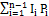with conditions:

Pc+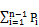=1 and P12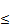Pn-1Pc.

Make fuzzy values of these parameters’ values to get fuzzy inputs to enter some fuzzy logic controllers to make decision based on some fuzzy inference rule sets whether transfer this RREQ to network or not.

c)  In every destination node, after processing for deciding this destination node can be on the route. If this node can belong to this route, it will calculate some parameters based on parameters’ values received from all nodes of the route which are fuzzed and entered fuzzy logic controller to make decision to select this route for transmitting data.

4.2. Evaluate Route at Intermediate Node

4.2.1. Choose Route Based on Fuzzy Logic

1)  Evaluate remaining power: if node has reserved power less than a regulated threshold then it is not selected, therefore this RREQ will be discarded.

2)  Evaluate energy consumption (J): Energy consumption is depended on some factors:

a)  Received signal strength;

b)  The size of data has to be transmit through this node;

c)  The number of node access channel concurrently (channel contention);

d)  Distance between two nodes (current node and sender of this RREQ), it is measured based on sender’s coordinates.

In which consumption energy can be calculated by MTPCR protocol (Minimum Transmission Power Consumption Routing). If J is small means network will operate consistency, J is more than one defined threshold then can not use this node for the route, discard RREQ.

3)  Length of the queue at every node: The smaller this value, the more route can work well.

4)  The delay in a node: The smaller this value, the more route can work well.

Every parameter I has defined values domain [Imin, Imax], in which Imin, Imax are minimum value and maximum value of corresponding parameter. If parameter value is not in this domain, assume do not process this value. Define the rate, therefore R belongs to the range [0, 1], divide these parameters into two groups :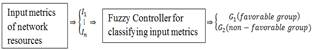a) The first group (G1): with these parameters, the larger this R, the more network can work well;

b) The second group (G2): with these parameters, the smaller value of R, the more network can work well.

Evaluate the probability to select current node on this route by one of two methods:

a)  Method 1: based on probability, the multiplication of the rates of all parameters belong to a group G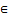{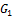,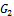}, give a estimating rate: FG=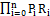, in which Pi is probability applying Ri on the result and satisfy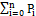=1;

b)  Method 2: based on fuzzy logic system as the following sections.

4.2.2. Establish Inference Rule Set

At first combining fuzzy input values into logical predicates. Make inference rule set to combine some logical predicates of some different metrics to make one logical predicate. For example: if remaining power of node is VL and energy consumption is VL then estimation of route through this node is HH.

Apply one of two methods: i) Set up fuzzy inference rule set for each summary metric FG, then combine two rule sets to make final inference rule set; ii) for each parameter I, calculate one metric Ri belongs to range [0,1]. At that time, set up the inference rule set, combine gradually two sets with each other, have final rule set for making decision.

Simulate by Pseudo code: have two sets of rules:

R1={R11, R12, …, R1n},

R2={R21, R22, …, R2m},

the result rule set by combining these two rule sets is Rq set:

a)  initialization by assigning: Rq={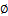}.

b)  For each rule R1i of set R1 execute (c);

c)  For each rule R2j of set R2 execute (d);

d)  Rq=Rq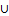{R1i& R2j};

e)  The result of algorithm is the set Rq has (n*m) rules.

4.2.3. Establish Membership Functions Of Input And Output Parameters

a)  Each parameter Mi will be fuzzed, into the rate Ri with value belongs the range [0, 1].

b)  Each parameter is evaluated by some membership functions  are: VL, L, M, H, VH. These membership functions will evaluate the membership of the fuzzy parameters Ri. These functions mean Very Low, Low, Medium, High, Very High. Membership function evaluates the ability x belongs the set F denoted by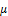F(x), in which x is value of estimated parameter that is bounded by the range [0, 1], F is a set of real values belongs to the range [0, 1] as defined in Fig. 4.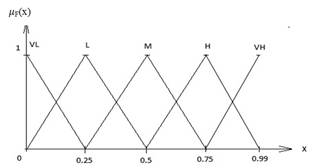Fig. 4. Membership functions for Energy consumption and Remaining power of node .

c)  Establish fuzzy inference system in  to estimate two parameters consisted of remaining power and energy consumption of current node in order to balance these two factors in evaluating the route for data transmission. According to results of calculating two rates above R1, R2 to estimate ability to use current node for the route. Depend on the membership functions of each parameter to build combined rule table to estimate two parameters as the following table:

Table. 1. Define rule base for fuzzy logic system .

 Estimation of route through node Energy consumption VL L M H VH Remaining power of node VL HH HM HL MH MM L HM HL MH MM ML M HL MH MM ML LH H MH MM ML LH LM VH MM ML LH LM LL

d)  In this Fuzzy Logic Controller of , there are 25 rules have been defined. The first rule is:

(R1) if remaining power of node is VL and energy consumption is VL then estimation of route through this node is HH; …;

(R25) if remaining power of node is VH and energy consumption is VH then estimation of route through this node is LL.

Therefore the purpose of a Fuzzy Inference System (FIS) is mapping from fuzzy inputs to outputs by the rule set.

e)  From above table of rule set, can make the membership function for fuzzy output variables.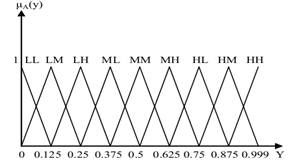Fig. 5. Membership functions for Fuzzy Output variable (Route_quality) of FIS.

Process for getting real values from fuzzy values is the process that performs:

1)  Based on the inference rules to get fuzzy output sets for membership functions respectively.

2)  Convert fuzzy output set into a value of result in number format;

3)  This number can be used to get decisions based on some membership functions for fuzzy output variable.

f)   Calculate fuzzy output result of FIS:

1)  With one input variable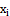: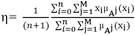, (n+1) is number of times of test, M is number of inference rules,is membership value of fuzzy membership functionfor input value;

1)  With n input variables: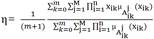, (m+1) is number of times of test,

M is number of inference rules,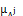(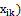is value of membership functionwith fuzzy input value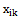.

Depend on results of FIS, will decide whether select current node belongs to the route or not.

4.3. Evaluate Route In Destination Node

In destination node, after estimate this node as a mediate node as in section (4.2) above, have to evaluate entire route [4.2] as below:

a)  Choose the route by hop count: If choosing route depends only on hop count of the route, for example, check if length of route is less than regulated threshold. If this route does not satisfy the condition, it is discarded.

b)  Choose the route by links’ bandwidth: It can depend on calculated links’ bandwidth, the route’s bandwidth can be calculated by . If the route’s bandwidth satisfies the bandwidth requirement then the route’s information will be send to the source node by RREP packet.

c)  Choose route based on fuzzy logic:

According to , for each detected route (R), build a vector (V) with k dimensions (equal to number of input metrics) to measure fuzzy values for these metrics on this route. Assume there are n detected routes: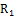,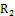, …,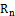, with n calculated resource vectors: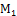,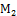, …,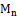and n path vectors: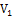,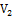, …,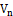for these routes respectively.

Calculate fuzzy cost for each metric i (i=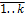) in each route j (j=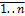):

1)  If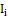in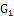: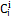=(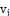(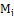()))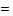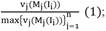2)  Ifin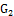:=((()))3)  From (1) and (2) formulas, make the algorithm for calculating fuzzy cost for each route as , assume C[1..n][1..k] is two dimensions matrix for calculating fuzzy cost for each dimension in each route, cost[1..n] is one dimension matrix for calculating fuzzy cost for n routes:

4)  Algorithm for calculating C[1..n][1..k]:

For each i from 1 to n (number of route) do:

For each j from 1 to k (number of network resources) do:

If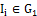then=((()))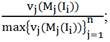Else=((()))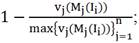Algorithm for calculating cost[1..n] based on C[1..n][1..k]:

For each i from 1 to n (number of route) do:

Begin

cost_tmp = 0;

For each j from 1 to k (number of network resources) do:

cost_tmp = cost_tmp +;

cost[i] = cost_tmp / k;

End;

So cost[i] will be in the range [0,1], apply fuzzy output membership functions to get the decision to accept the route.

Otherwise route can be predicted by a delivery probability  before using it. The delivery probability is performed during temporary disconnections. According to  evaluating each metric at a time so that optimum value of a higher priority goal is never degraded by a lower priority goal.

4.4. Choose The Route In The Source Node

In the source node, can receive more than one RREP packet from one destination node. Because all route have been evaluated in destination nodes, so can choose the best route to transfer data, or split data into many smaller parts that are transmitted on many established routes.

a)  Select route by hop count: choose route that has minimum hop count to transfer data.

b)  Select route by route’s bandwidth: choose route that has maximum bandwidth to transfer data.

c)  Select multipath route with guaranteed QoS: in the case the data need to be transferred is very big, split this data into many smaller packets then transfer these packets on multi-paths with guaranteed bandwidth or hop count. Several packets received in destination nodes will be combined to make an original packet.

4.5. Package Priority Scheduling Based on Fuzzy Logic

For transmitting data effectively and reflecting real situation, especially in service based routing , have to schedule data with different priority levels. There are some algorithms for scheduling packages , in this paper present the package scheduling by fuzzy logic. Build fuzzy controller with some input metrics of received package and process these inputs to get one output result that is the priority to transfer this package. Based on this priority to put this package into respective queue for transmitting. In addition with some metrics mentioned in , this paper mentions service name for incoming message if this message is belonged to service based routing protocol. For example, if message is of network monitor service that has high priority.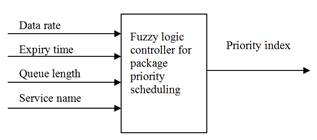Fig. 6. Package priority scheduling based on fuzzy logic.

Fuzzy controller processes based on four metrics consisted of three metrics of package level and one at node level: i) package level: data rate, expiry time, service name; ii) node level: queue length to get priority index by some processes. At first can do by one of two methods:

a)  Method 1: Categorize data by service class into some priority queues, each queue stores packages of certain range of priority;

b)  Method 2: Assign each service with a number in the range [0, 1] for assessing by fuzzy controller.

If doing by method 1, next calculate particular priority for each package in each queue based on three remaining metrics according to . Otherwise build fuzzy logic rules and inferences based on four metrics to get priority index.

4.6. Some Security Problems

By using a pair of packets RREQ and RREP to detect routes based on processes and transitions in mediate nodes. Therefore the ability to be attacked by black hole type that is very easily happened when receiving RREQ packet. Attacker is the node that will not process and forward RREQ but create RREP packet to send back to the source node. The source node will accept the routes of all received RREPs, so all routes will be passed through this node and data will be sent to it. Leading to reveal and loss data, system will be in congestion state. It need to build a system to detect attacks based on fuzzy logic to prevent . The fuzzy logic function accepts an input parameter that is the rate of packets which are transited through the node. This rate is computed by formula: packet_forward_ratio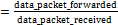. This fuzzy system will compute fuzzy output based on frequently inputs that are measured by every node. The fuzzy result is called (Fidelity_level) denoted by FL. The value of FL will be sent to a monitor node for detecting attackers:

a)  If FL=0: can conclude that node is completely valid;

b)  if FL=1: can conclude this node is completely invalid;

c)  if FL is in the range (0, 1), conclude whether node is back hole or not based on a threshold value F.

5. Cluster Network Based on Fuzzy Logic

Cluster network based on fuzzy logic  help to reduce cost of routing and load of network, use bandwidth effectively. Based on clustering the routing can be process hierarchically, so the network is easily expanded.

5.1. Clustering Model

Dividing a large network into some smaller clusters, each cluster will choose a manager node that manages all resources in this cluster and communicates information with other manager nodes of another clusters. This clustering model help to reduce number of nodes relating to the entire network routing, reduce network congestion, throughput and load.

5.2. Method of Implementation

This clustering model uses computation results of fuzzy logic functions at every node on network with its neighbor nodes. The input power parameter Ei is measures continuously from signals received at different times Ti in time interval T of every node to get the result metric E for clustering network. The node has maximum value Emax that will be chosen the manager of cluster (M). Some neighbor nodes (N) of M that have EN which is more than a predefined threshold value (ET) and less than E will be chosen in one cluster with node M so that it satisfies formula: E=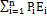and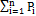=1, in which n is number of neighbor nodes.

Cluster network based on mobility characteristic as presented in , so in this paper, after applying above method, if a set of nodes found that have the same level of input power. Choose node that have minimum mobility to be the cluster head.

6. Simulation and Evaluation

Based on these analyses above, in this paper, make some modules to simulate the fuzzy logic controller and calculate fuzzy values based on parameter values of some metrics. Code is written in C#:

a)  Code for fuzzy logic controller:

Public class cFuzzyLogicController

{

Public List<cFuzzyRule> fuzzy_rule_base;

Public cDefuzzification defuzzification;

Public List<cMemberShipFunction> membership_list;

Public cFuzzyLogicController()

{

fuzzy_rule_base = new List<cFuzzyRule>();

membership_list = new List<cMemberShipFunction>();

defuzzification = new cDefuzzification();

}

Public void addrule(double lb, double ub, string res, string para)

{

defuzzification.addrule(lb, ub, res, para);

}

Public void add_membership_func(string mem_func_name, double dlocal_min, double dlocal_max, string para_name)

{

cMemberShipFunction member_func;

member_func = membership_list.Find(memtmpfunc => memtmpfunc.function_name == mem_func_name &&

memtmpfunc.parameter_name == para_name);

if (member_func == null)

{

member_func = new cMemberShipFunction();

member_func.giatri_bd = dlocal_min;

member_func.giatri_kt = dlocal_max;

member_func.function_name = mem_func_name;

member_func.parameter_name = para_name;

}

}

Public void membership_func_add_point(double begin_point, double end_point, ref cMemberShipFunction member_func)

{

Pointcur_point = new point(begin_point, end_point);

}

Public double calculate_fuzzy_result(double member_value)

{

Double dFuzzy_result = 0;

Double dFuzzy_tuso = 0;

Double dFuzzy_mauso = 0;

for (int i = 0; i<membership_list.Count; i++)

{

dFuzzy_tuso += member_value * membership_list[i].get_membership_value(member_value);

dFuzzy_mauso += membership_list[i].get_membership_value(member_value);

}

if (dFuzzy_mauso != 0)

{

dFuzzy_result = dFuzzy_tuso / dFuzzy_mauso;

}

Return dFuzzy_result;

}

}

b)  Code for getting real values from fuzzy cost values to decide appropriate route:

Public class cDefuzzification

{

Public List<cFuzzyRule> fuzzyrulebase;

Public cDefuzzification()

{

fuzzyrulebase = newList<cFuzzyRule>();

}

Public void addrule(double lb, double ub, string res, string para)

{

try

{

cFuzzyRule fr;

if (fuzzyrulebase.Count> 0)

{

fr = fuzzyrulebase.Find(cur_rule =>cur_rule.lowerbound == lb &&

cur_rule.upperbound == ub && cur_rule.fuzzyresult == res &&

cur_rule.fuzzyparameter == para);

if (fr == null)

{

fr = newcFuzzyRule(lb, ub, res, para);

}

}

}

catch

{

}

}

Public string defuzzification(double fuzzyvalue)

{

String ketqua = null;

try

{

fuzzyrulebase.Sort(comparerule);

for (int i = 0; i<fuzzyrulebase.Count; i++)

{

if (fuzzyrulebase[i].lowerbound<= fuzzyvalue && fuzzyrulebase[i].upperbound>= fuzzyvalue)

{

ketqua = fuzzyrulebase[i].fuzzyresult;

break;

}

}

}

catch

{

}

Return ketqua;

}

Private int comparerule(cFuzzyRule r1, cFuzzyRule r2)

{

return r1.lowerbound.CompareTo(r2.lowerbound);

}

}

c)  According to , compare results of protocol performance of On Demand Multicast Routing Protocol (ODMRP) with the case using fuzzy logic controller and the case not use it in some network conditions. Compare join queries, energy consumption, throughput and radio collisions with node speed, different radio range of node.

1)  Following are diagrams that present the throughput of ODMRP protocol with varying node speed in different radio range of node: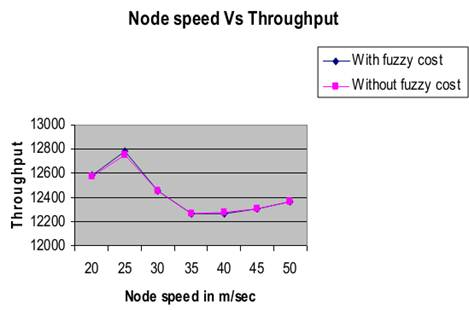Fig. 7. Node mobility versus throughput in transmission range 283 m.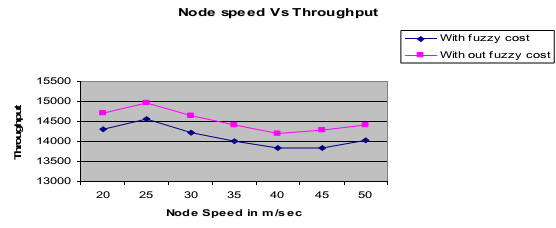Fig. 8. Node mobility versus throughput in transmission range 377 m.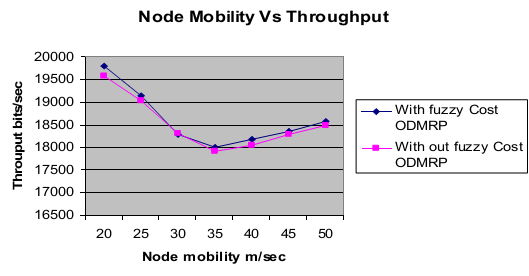Fig. 9. Node mobility versus throughput in transmission range 503 m.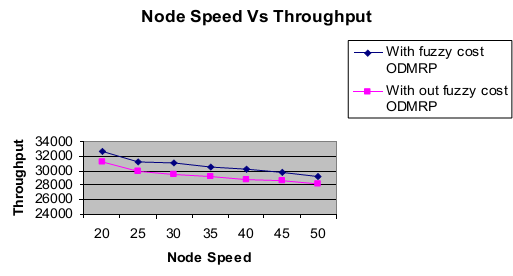Fig. 10. Node mobility versus throughput in transmission range 671 m.

2)  Following are diagrams that present the radio collisions with node speed in different radio ranges of node: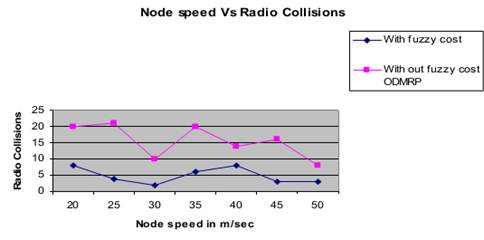Fig. 11. Node speed versus radio collisions in transmission range 377 m.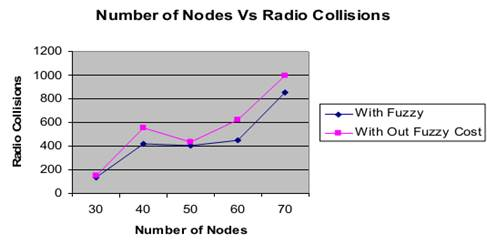Fig. 12. Node speed versus radio collisions in transmission range 377 m.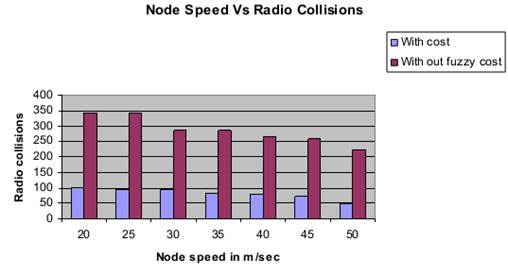Fig. 13. Node speed versus radio collisions in transmission range 503 m.

d)  Evaluation result is presented in Fig. 14, that is result by calculating the end to end delay that is time to transfer package from source to destination node, when number of node changes in two cases with fuzzy package scheduling and without it.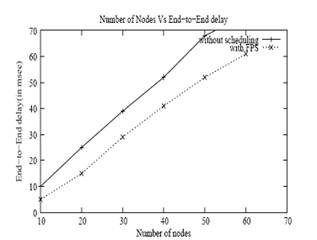Fig. 14. End to end delay Vs number of nodes .

References

1. P.Venkateswaran, MousumiKundu, SAMEER, KolkataCentre, Srishti Shaw, Kanika Orea, R.Nandi, "Fuzzy Logic-Based Mobility Metric Clustering Algorithm for MANETs", JadavpurUniversity, India, July-September 2011.
2. EktaKamboj, Harish Rohil, "Detection of Black Hole Attack on AODV in MANET Using Fuzzy Logic", Dept. Of CSA, CDLU Sirsa, India, 2011.
3. Nguyen Thanh Long, Nguyen DucThuy, Pham Huy Hoang, "Research on Innovating, Evaluating and Applying Multicast Routing Technique for Routing messages in Service-oriented Routing", Springer, ISBN: 978-1-936968-65-7, Volume Number 109, 2012.
4. Http://vi.wikipedia.org/wiki/Logic_m%E1%BB%9D.
5. S.Abirami, V.Bhanumathi, R.Dhanasekaran, "A Balanced Approach for Power Aware Routing in MANET using Fuzzy Logic", ICON3C 2012.
6. M.Marimuthu, A.Kannammal, "Fuzzy Cost based Power Aware QoS Routing Protocol with Mobility Prediction in Mobile Ad Hoc Networks", International Journal of Computer Applications (0975 – 8887), Volume 58– No.15, November 2012.
7. Http://voer.edu.vn/bai-viet/khoa-hoc-va-cong-nghe/suy-luan-voi-cac-tap-mo-fuzzy-logic.html.
8. Lada-OnLertsuwanakul, Faculty of Mathematicsand Computer Science, Fern Universität in Hagen, Universitätsstraße 27 –PRG, 58084Hagen, "Fuzzy Logic Based Routing in Grid Overlay Network".
9. A Naga Raju, Dr S Rmachandram, Head & Associate Prof in I.T,Kamala Institute of Tech & Science, "FUZZY COST BASED MULTIPATH ROUTING FOR MOBILE AD-HOC NETWORKS", Journal of Theoretical and Applied Information Technology, © 2005 - 2008 JATIT.
10. Bavithiraja, S.V.M.G. and R. Radhakrishnan Department of CSE,CMS College of Engineering and Technology, Coimbatore-32, India Sri Ramakrishna Engineering College, Coimbatore-22, India, "Efficient Broadcasting in Mobile Ad Hoc Networks Using Context Aware Adaptive Routing Protocol during Network Partitions".
11. Krishna. G. Deshpande, Department of E&CE, Basaveshwar Engineering College, Bagalkot, Karnataka, INDIA, "Agent Approach QoS Routing in Manet Based on Fuzzy Priority Scheduler".

 Contents 1. 1.1. 1.2. 2. 3. 3.1. 3.2. 3.3. 3.4. 4. 4.1. 4.2. 4.3. 4.4. 4.5. 4.6. 5. 5.1. 5.2. 6.
Article ToolsAbstractPDF(489K)